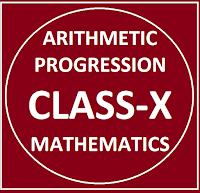## Posts

Showing posts from January, 2019

### Common Errors in Secondary Mathematics

Common Errors Committed  by the  Students  in Secondary Mathematics   Errors  that students often make in doing secondary mathematics  during their practice and during the examinations  and their remedial measures are well explained here stp by step.  Some Common Errors in Mathematics

### Conic Sections /Circle & Parabola/Chapter-11/Class-XICONIC SECTION The sections of the double napped right circular cone with the plane are called conic sections. eg:- circle, ellipse, parabola and hyperbola etc. all are the examples of conic sections. APPLICATIONS:- These curves have wide applications in the field of planetary motion, design of telescope, antenas, reflectors of flash light and automobile headlights. Vertex of the double napped cone separate the cone into two parts each part is called Nappes. α (alpha) is the angle made by the generator with the x-axis. Maths Conic Section Part 1 Class 11 NCERT-cbse mathematics DIFFERENT TYPES OF CONIC SECTIONS:- CIRCLE:-   When β(beta) = 90, then intersection of cone with plane is called circle. ELLIPSE:- When α<β<90 then intersection of plane and cone gives an ellipse. PARABOLA:- When α= β then intersection of plane and cone gives parabola. HYPERBOLA:- When 0<β<α then the intersection of plane and cone gives a hyperbola. Maths Conic

### CBSE Class 10 Maths Formulas Chapter-05 | Arithmetic ProgressionARITHMETIC PROGRESSION Class 10 Chapter 5 &  Class 11 Chapter 9 Basics and all formulas related to the Sequence and series (Arithmetic Progression) useful for classes X & XI strictly according to the CBSE board

### Inverse Trigonometric Functions Chapter 2 Class 12INVERSE   TRIGONOMETRIC   FUNCTIONS Maths formulas based on Inverse trigonometric functions chapter 2 class 12, useful and important maths formulas on Inverse Trigonometric Functions Note: Click here to open the  Assignment on Inverse Trigonometric Functions  Chapter 2 Class 12 Before we start the Inverse Trigonometric functions we should know the trigonometric functions class 11 chapter 3 . First of all students should learn all the formulas of trigonometric functions then start this chapter. Inverse Trigonometric Functions CBSE Syllabus For Inverse Trigonometric Functions Chapter 2 Class XII  Definition, range, domain, principal value branch, Graphs of inverse trigonometric functions, Elementary Properties of Inverse Trigonometric Functions 1.) In this section we discuss about the principal value 2.) Domain, and range of Inverse Trigonometric Functions 3.) Properties of Inverse Trigonometric Functions. 4.) Graph of Inverse of Trigonometric Functions. ******